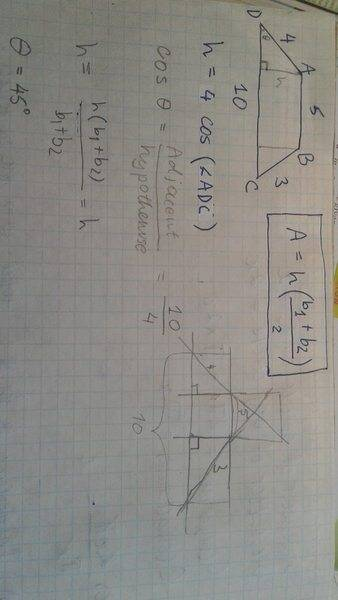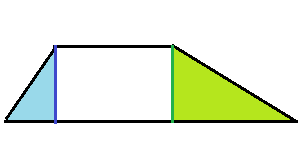# Finding height and area of trapezoid from its legs and bases

• ISamson

#### ISamson

Gold Member
Hello.
I am wondering how I can find the area of a trapezoid from its two legs and bases.
My problem:
ABCD is a trapezium with AB parallel to CD such that AB = 5, BC = 3, CD = 10 and AD = 4. What is the area of ABCD?
If we trace a straight line from A down parallel to the height of the trapezium, we get a right - angle triangle. From this triangle we can find the height by calculating the cosine of ∠ADC, then find the area by the Area of Trapezium formula. This should solve the problem, right?
Sources:
http://www.analyzemath.com/Geometry/trapezoid_solve.html
http://www.bbc.co.uk/schools/gcsebitesize/maths/geometry/furthertrigonometryhirev2.shtml
https://www.mathsisfun.com/sine-cosine-tangent.html

I am a high school student. Unfortunately, still did not learn anything about cos, sin and tan, but I would like to know if I am on the right path with this problem.

Thank you.
EDIT: and also, do I have to solve this using cos and trigonometry, or can I use another method if it is more simple?

Last edited:
I moved the thread to our homework section.
ISamson said:
From this triangle we can find the height by calculating the cosine of ∠ADC
You only know two sides of the triangle ADC. How would you find the height?

It is possible to solve this problem without trigonometry, but there is a trick you have to find.

•ISamson
mfb said:
You only know two sides of the triangle ADC.
The hypothenuse - I know.
I also know all of the DC segment, however I am not sure what the DC-base side of the triangle is exactly. Do I?

mfb said:
but there is a trick you have to find.
So, I can find a trick (is it not too hard? :)

ISamson said:
however I am not sure what the DC-base side of the triangle is exactly. Do I?
That is difficult to figure out directly.

Did you draw a sketch?
ISamson said:
So, I can find a trick (is it not too hard? :)
I don't think it is hard, but that is subjective.
Think of how the trapezoid changes if you change both AB and CD by the same amount. What changes, what stays the same?

mfb said:
Spoiler
The area changes, the length of the AB and CD changes, the length of the legs does not change, the height does not change, all the angles do not change.

Yes, I thought about it for a long time, and drew a sketch.#### Attachments

I don't know what b1 and b2 are, but the angle is not 45 degrees.
The formula you use for the angle only works if you use a rectangular triangle, and the cosine of something cannot be 10/4 which is larger than 1.
ISamson said:
The area changes, the length of the AB and CD changes, the length of the legs does not change, the height does not change, all the angles do not change.
Good. If you want to find the height or angles you can change both lengths together. Do you find a change that makes the diagram much simpler to study?

ISamson said:
The hypothenuse - I know.
∆ACD is not a right-angled triangle.

ISamson said:
I am a high school student. Unfortunately, still did not learn anything about cos, sin and tan, but I would like to know if I am on the right path with this problem.

Thank you.
EDIT: and also, do I have to solve this using cos and trigonometry, or can I use another method if it is more simple?
You certainly learned some famous law concerning right triangles. And you can draw two of them, with equal heights.#### Attachments

•ISamson
Have you done the question?

ISamson said:
Yes. Why?
View attachment 228941
Can you see that?
I can see. I just didnt know whether you did an you were stuck. Good Job
E

•ISamson
Elbraido said:
I can see. I just didnt know whether you did an you were stuck. Good Job
E
Thanks

Here is how I would have solved it: Draw a parallel to BC going through A. It crosses CD at point E. We know ED=AB=5, therefore DE=DC-EC=5. That's basically the idea "make the trapezoid 5 shorter" I was hinting at.
The triangle DAB has side lengths DA=4, AE=3, ED=5. It is a right triangle with the right angle at A. This is "by accident", but I think it has been done deliberately. We can express its area in two different ways: 1/2 (DA)(AE) = 1/2 (ED) h. Therefore, h=(DA)(AE)/(ED) = 4*3/5 = 12/5.
This leads to the total area A=12/5 (10+5)/2 = 18.